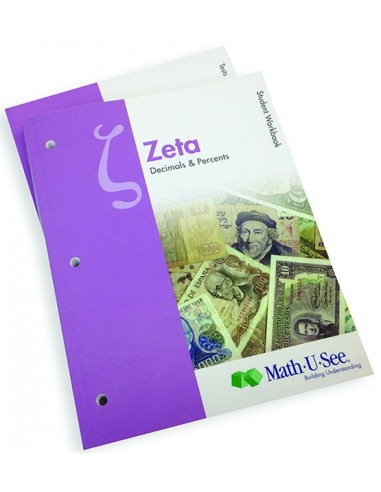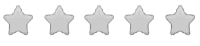See inside this book

# Math-U-See Zeta Student Pack

\$42.00
No tax
Demme Learning
Math
6
P-MA06-62Leave a Review

#### Description

New! Math-U-See Zeta - Student Pack

Math-U-See is a complete, skill-based, multi-sensory homeschool math curriculum for grades K-12 that?s designed to teach students skills in a logical sequence.

The Zeta Student Pack is for those who already have the other required components for the course. It contains the Student Workbook with lesson-by-lesson worksheets, systematic review pages, and Application & Enrichment pages as well as Zeta Tests.

Zeta extends the student?s concept of place value to the right of the decimal point. Students learn to complete core operations with decimals. The connection between fractions and decimals is presented.

Major Concepts and Skills Include:

Expanding understanding of place value from positive powers of ten to include decimals Fluently adding, subtracting, multiplying, and dividing multiple digit decimals using place-value strategies Solving real-world problems with decimals and percentages Understanding the metric system and converting from one unit of measure to another

Understanding and simplifying exponents Understanding negative numbers and representing them on the coordinate plane Using properties of operations to simplify and evaluate algebraic expressions Interpreting and graphing relationships between dependent and independent variables Understanding of plane geometry & geometric symbols Using ratio reasoning to solve problems